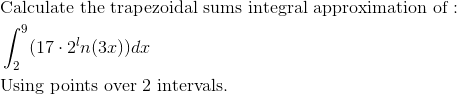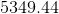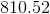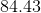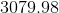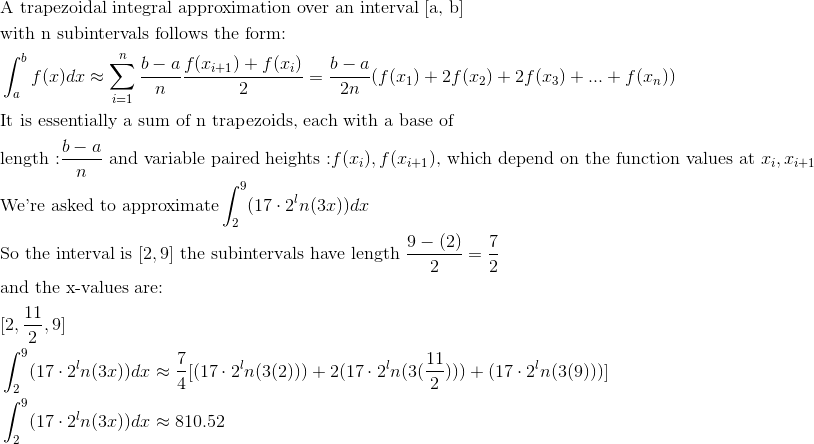AP Calculus AB : Trapezoidal sums

Example Questions

← Previous 1 3 4 5

Example Question #1 : Trapezoidal Sums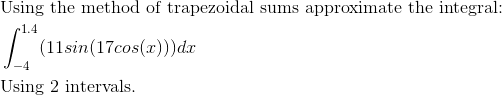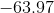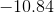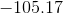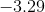Explanation: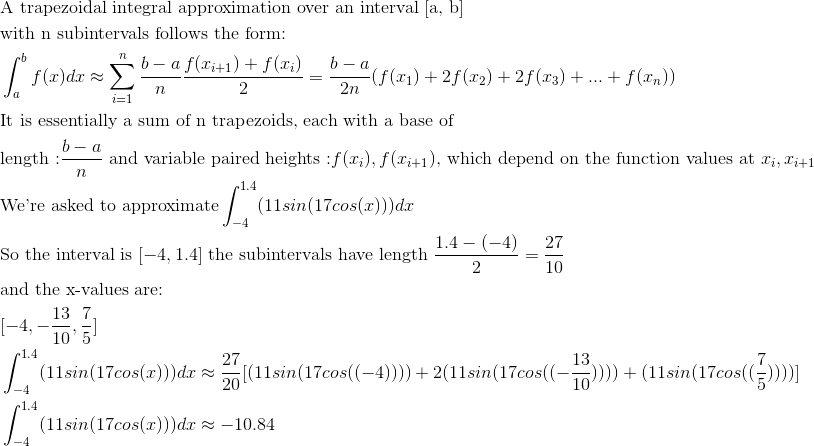Example Question #2 : Trapezoidal Sums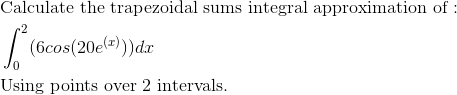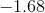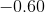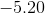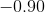Explanation: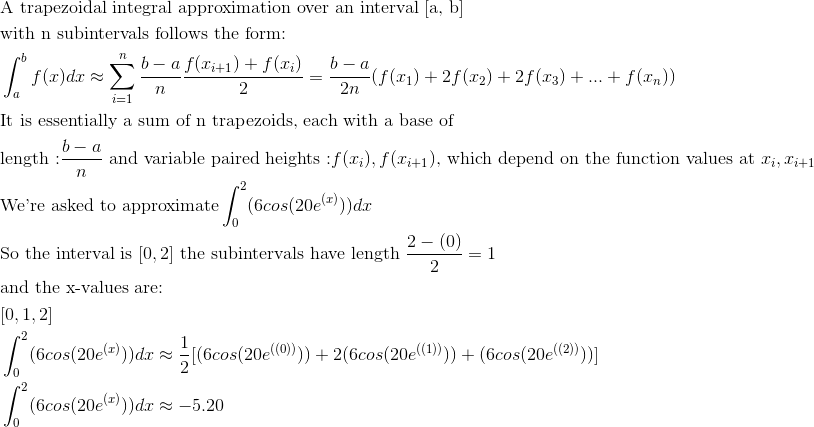Example Question #3 : Trapezoidal Sums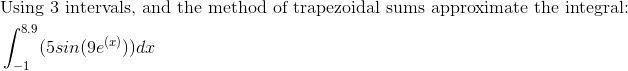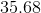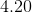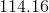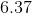Explanation: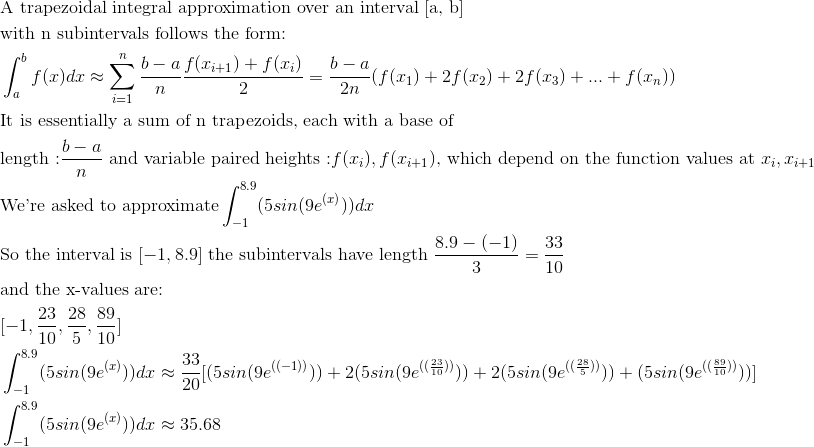Example Question #4 : Trapezoidal Sums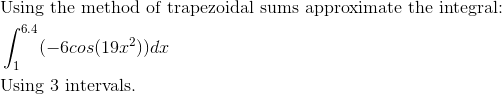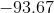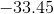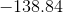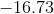Explanation:Example Question #5 : Trapezoidal Sums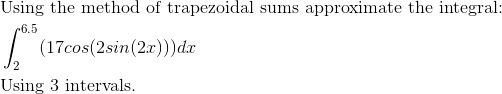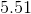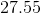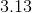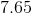Explanation: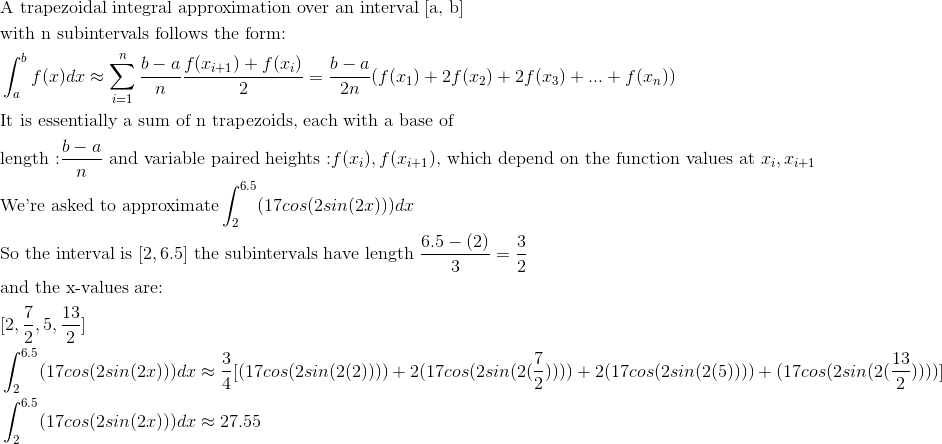Example Question #6 : Trapezoidal Sums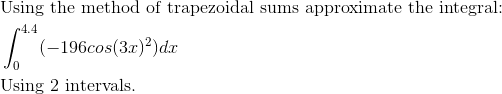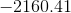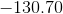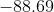Explanation: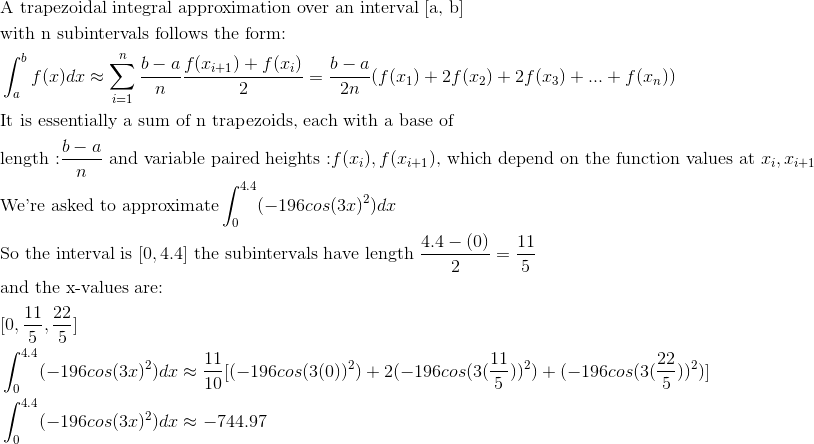Example Question #7 : Trapezoidal Sums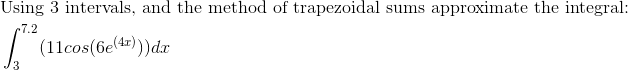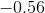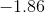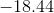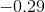Explanation: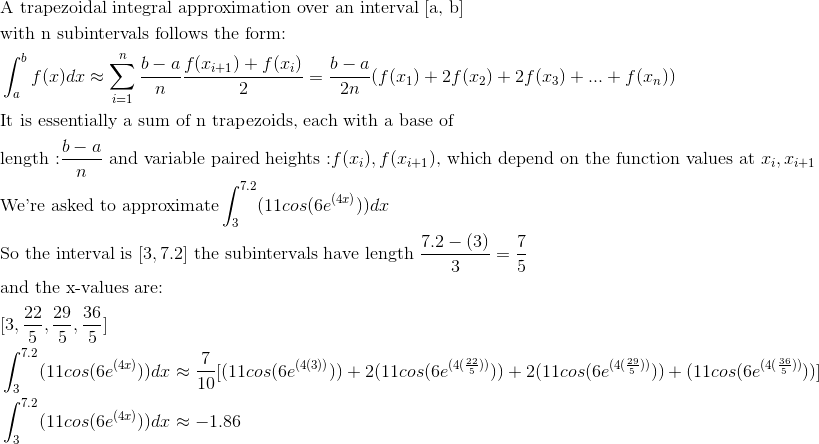Example Question #8 : Trapezoidal Sums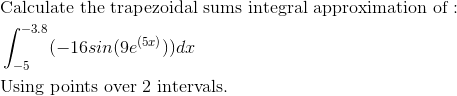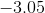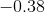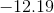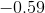Explanation: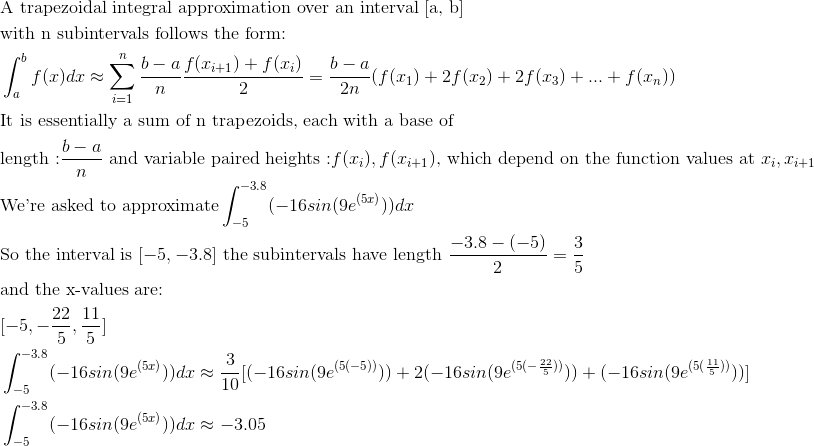Example Question #9 : Trapezoidal Sums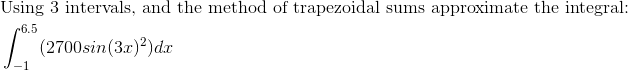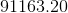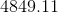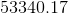Explanation: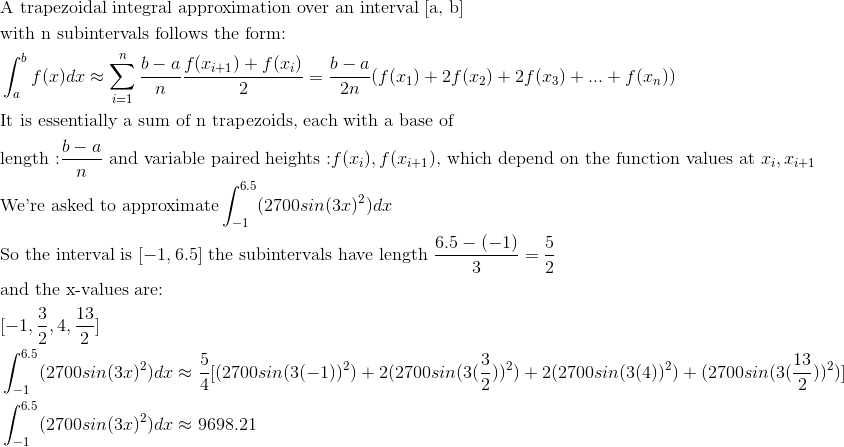Example Question #10 : Trapezoidal Sums